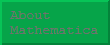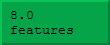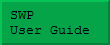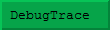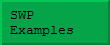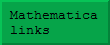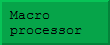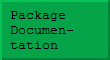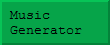## Introduction

### Symbolic algebra and calculus.

Mathematica can perform all the usual calculus operations – such as integration, differentiation, series, limits, etc. – and it can often obtain answers where you or I would give up. This is because it can, of course handle extremely large calculations, but also because it 'knows' about many special functions. For example: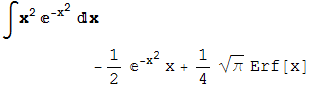### Symbolic programming.

Mathematica is also a powerful general programming language. This means that in addition to using the built-in symbolic operations – such as algebra and calculus, it is possible to write extensions to cover areas such as Feynman integrals or various aspects of abstract algebra (groups, rings, fields, etc.).

### Bignum integer arithmetic and arbitrary precision real arithmetic.

Mathematica does not have the concept of integer overflow – so familiar in other computer languages. A calculation such as 25 factorial evaluates exactly to 15511210043330985984000000, and calculations involving integers with hundreds of decimal digits can be easily performed. The largest size of integers are limited only by memory constraints and the increasing computational costs of arithmetic. This feature is vital in computer algebra, where coefficients can become extremely large in intermediate calculations, even if the final result is smaller.

Since most integers will fit into 32-bits, Mathematica stores these in a more efficient way, switching to the indefinite length format when overflow would otherwise occur.

In much the same way, real (floating point) data is normally stored in 64-bit IEEE format, but it is easy to specify floating point quantities of much higher precision. Obviously, some applications benefit directly from this extra precision. However it can also be used in rather novel ways. For example, consider the following well known identity: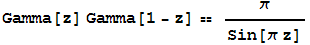Suppose we want to check this numerically. We start by creating a 100-digit random number:and substitute this into a form of the identity: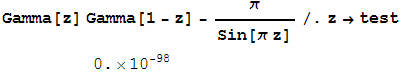Obviously, such a calculation does not constitute a mathematical proof, but it supplies a very strong hint that an identity is true, and it can avoid much wasted time trying to prove identities that are false!

This general idea has been taken much further in the PSLQ algorithm which tries to find suitable integers ai so that: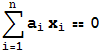This algorithm performs an efficient search for suitable integers, and if the xi are high-precision quantities, the result, if found, is almost certainly true. Totally new identities have been found in this way and subsequently proved.

Please note that the D.H.Bailey who is refered to in the above link about the PSLQ algorithm is not me, and is not related to me.

### Programmable frontend

Some of the results on this page already hint at the power of the Mathematica frontend (the part of Mathematica that handles notebooks and interacts with the user) to display mathematics in a congenial way. What is even better, is that (with a little effort) it is possible to program the frontend to understand and display your favourite notations. This opens up a whole range of applications, such as the coloured algebra concept.